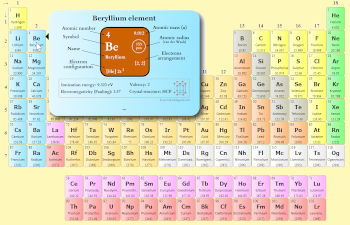# 5 Reasons Why Periodic table is Divided into Groups (Simple)

The Periodic table is divided into groups for the following reasons;

• To classify the elements according to their similar properties.
• By dividing the elements in groups, it becomes very easy to study them.
• By dividing the Periodic table in groups, you can easily make a group wise comparison of elements.
• You can easily find the electron shells by knowing the position of elements in groups.
• You can also find the valence electrons by knowing the group wise position of elements.

This was the overview.

But I have given a plain english explanation of the above points. (See below).See how this Interactive Periodic Table helps you

• You can effortlessly find every single detail about the elements from this single Interactive Periodic table.
• You will get the detailed information about the periodic table which will convert a newbie into pro.
• You will also get the HD images of the Periodic table (for FREE).

Visit ➢ Periodic table

## Reason #1: To classify the elements according to their similar properties

Look at the above image.

Imagine that you have to find the element which is non metallic in nature, then how will you find it from the above image?

It’s very difficult to find, isn’t it?

Hence in order to find the element easily, the Periodic table was developed by the scientists and the elements were classified according to their similar properties.

On today’s periodic table, you can easily find the metals on the left side, Nonmetals on the right side and Metalloids in between them.

## Reason #2: By dividing the elements in groups, it becomes very easy to study them

There are just a few elements. But what if you have to study all the 118 elements?

If all the elements are randomly placed here and there, then obviously it will be difficult for you to study all of them.

So in order to make the study easy, the elements of the Periodic table are divided into 18 groups.

Elements of each group on the Periodic table have different physical and chemical properties.

So if there is a group wise classification in Periodic table, then the study of these elements would become very easy.

## Reason #3: By dividing the Periodic table in groups, you can easily make a group wise comparison of elements

Let me ask you a question.

How does lithium differ from fluorine?

How does aluminum differ from copper?

How does calcium differ from silicon?

It’s difficult to answer these questions.

But if you know the position of these elements in periodic table, then it would be very easy to make such comparisons.

Hence grouping of elements is necessary on periodic table.

## Reason #4: You can easily find the electron shells by knowing the position of elements in groups

Yes, by knowing the position of elements in the groups, you can also find the number of electron shells.

Let me ask you a question,

How many shells does lithium have?

How many shells does chlorine have?

How many shells does oxygen have?

You may not be knowing the answers of the above questions.

But if you know the positions of these elements in periodic table, then you can easily find the number of shells present in them.

For example in group 1, lithium element lie at the 2nd position.

Hence, the lithium element has 2 electron shells.

Similarly, in group 17, chlorine element lies at the 3rd position (or in 3rd period).

Hence, chlorine element has 3 electron shells.

For more information, see the image of Periodic table with electrons per shell. (This Periodic table has the shell diagram of all the elements mentioned on the Periodic table itself.)

## Reason #5: You can also find the valence electrons by knowing the group wise position of elements

First of all, valence electrons are nothing but the number of electrons present in the outermost orbit of an atom.

But wait a minute, before explaining the 5th reason, I want to ask you a question.

How many valence electrons does oxygen have?

How many valence electrons does neon have?

How many valence electrons does magnesium have?

It’s difficult for a newbie to answer this question.

But if you know the position of these elements, then you can easily find the valence electrons of these elements.

For example, magnesium lies in the second group.

Hence, it has two valence electrons.

Oxygen lies in group 16. So it has 6 valence electrons.

Similarly, neon is in group 18. So it has 8 valence electrons.

In this way, by knowing the group wise position of elements, you can easily find their valence electrons.

## Free Gift for you: Interactive Periodic Table

Let me tell you how this Interactive Periodic Table will help you in your studies.

1).
You can effortlessly find every single detail about the elements from this single Interactive Periodic table.

(For Interactive Periodic table, view on laptop/desktop for better experience. If you are on mobile device, then use a “Desktop site mode” to see interactive periodic table)

2). You will get the detailed information about the periodic table which will convert a newbie into pro.

3). You will also get the HD images of the Periodic table (for FREE).

Checkout Interactive Periodic table and download it’s high resolution image now (It’s FREE)